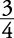## Slopes of Parallel and Perpendicular Lines

Parallel lines have equal slopes. Conversely, if two different lines have equal slopes, they are parallel. If two nonvertical lines are perpendicular, then their slopes are negative reciprocals (actually, opposite reciprocals) of one another, or the product of their slopes is –1. Conversely, if the slopes of two lines are opposite reciprocals of one another, or the product of their slopes is –1, then the lines are nonvertical perpendicular lines. Because horizontal and vertical lines are always perpendicular, then lines having a zero slope and an undefined slope are perpendicular.

##### Example 1

If line l has slope, then

1. any line parallel to line l will have slope ____, and

2. any line perpendicular to line l will have slope ____.

1.2.